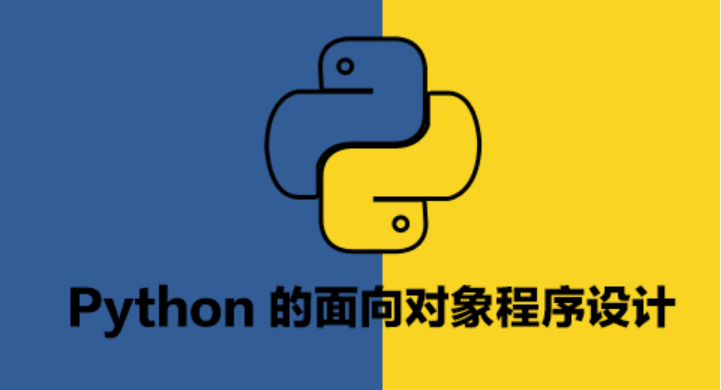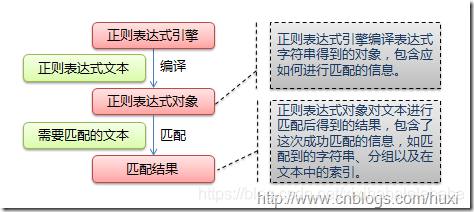# 正则表达式（三）：python re模块

+关注继续查看

python 提供 re 模块，来满足正则表达式的使用。在开始介绍 re 模块之前，首先说明一下两个小内容：

1. 转义字符 \

import re

reg = r'\\'
str = '\\'    #转义符号，str实际值为\

if re.match(reg,str):
print('match {}'.format(str))
------------------------

match \

1. Pattern、Match对象

• 在 python 中可以直接使用 re 模块生成 Match 对象，完成字符串处理。
示例：
import re

reg = r'abc'
str = 'abc'

mattern = re.match(reg,str)
print(mattern .group())
------------------------

abc

• 使用 re 模块生成 Pattern 对象，然后使用 Pattern 对象生成 Match 对象，完成字符串处理。
示例：
import re

reg = r'abc'
str = 'abc'

pattern = re.compile(reg)
match = pattern.match(str)
print(match.group())
------------------------

abc


# --------------------------------------------------------------------
# public interface

def match(pattern, string, flags=0):          #第一种方式
"""Try to apply the pattern at the start of the string, returning
a Match object, or None if no match was found."""
return _compile(pattern, flags).match(string)

def compile(pattern, flags=0):                #第二种方式
"Compile a regular expression pattern, returning a Pattern object."
return _compile(pattern, flags)

# --------------------------------------------------------------------
# internals

_cache = {}  # ordered!

_MAXCACHE = 512
def _compile(pattern, flags):
# internal: compile pattern
if isinstance(flags, RegexFlag):
flags = flags.value
try:
return _cache[type(pattern), pattern, flags]         #从缓存字典中查询
except KeyError:
pass
if isinstance(pattern, Pattern):
if flags:
raise ValueError(
"cannot process flags argument with a compiled pattern")
return pattern
if not sre_compile.isstring(pattern):
raise TypeError("first argument must be string or compiled pattern")
p = sre_compile.compile(pattern, flags)
if not (flags & DEBUG):
if len(_cache) >= _MAXCACHE:
# Drop the oldest item
try:
del _cache[next(iter(_cache))]
except (StopIteration, RuntimeError, KeyError):
pass
_cache[type(pattern), pattern, flags] = p         #添加到缓存字典中
return p


##### 下面列出 Pattern 对象中使用到的函数：

match(string, pos=0, endpos=-1) 在指定范围内，从指定的起始位置开始匹配，得到匹配对象则返回
search(string, pos=0, endpos=-1) 在指定范围内，从任意位置开始匹配，得到匹配对象则返回
findall(string, pos=0, endpos=-1) 在指定范围内，返回所有匹配结果构成的列表
finditer(string, pos=0, endpos=-1) 在指定范围内，返回所有匹配对象构成的迭代器
split(string, maxsplit=0) 按照指定的分割次数，返回分割得到的结果列表
sub(repl, string, count=0) 按照指定的替换规则和替换次数，返回替换后的结果
subn(repl, string, count=0) 按照指定的替换规则和替换次数，返回替换后的结果和替换次数构成的元组
• match 函数

match 函数返回指定范围内，从指定起始位置开始匹配到的对象。使用过程中只有三点需要注意一下：
1. 可以指定匹配的范围，默认范围是 0 到 len(str)
2. 匹配是从指定的起始位置开始的，也就是固定了开始位置
3. 匹配成功则返回，也就是只返回一个匹配对象

import re

reg = r'\d'
pattern = re.compile(reg)

str1 = 'a1b2c3'

match1 = pattern.match(str1)
match2 = pattern.match(str1,1)

print('match1 = {}'.format(match1))
if match1:
print('match1 matched = {}'.format(match1.group()))

print('match2 = {}'.format(match2))
if match2:
print('match2 matched = {}'.format(match2.group()))

match1 = None
match2 = <re.Match object; span=(1, 2), match='1'>
match2 matched = 1

• search 函数

search 函数返回指定范围内，从任意起始位置开始匹配到的对象。使用过程中只有三点需要注意一下：
1. 可以指定匹配的范围，默认范围是 0 到 len(str)
2. 匹配是从范围内的任意起始位置开始的，也就是不固定开始位置
3. 匹配成功则返回，也就是只返回一个匹配对象

import re

reg = r'\d'
pattern = re.compile(reg)

str1 = 'a1b2c3'

match1 = pattern.search(str1)

print('match1 = {}'.format(match1))
if match1:
print('match1 matched = {}'.format(match1.group()))

match1 = <re.Match object; span=(1, 2), match='1'>
match1 matched = 1


• findall 函数

findall 函数返回指定范围内，所有匹配结果构成的列表。使用过程中只有两点需要注意一下：
1. 可以指定匹配的范围，默认范围是 0 到 len(str)
2. 返回的是一个列表，元素是 str 对象，而非 Match 对象

import re

reg = r'\d'
pattern = re.compile(reg)

str1 = 'a1b2c3'

match1 = pattern.findall(str1)

print('match1 = {}'.format(match1))
for element in match1:
print('element = {}'.format(element))

match1 = ['1', '2', '3']
element = 1
element = 2
element = 3

• finditer 函数

finditer 函数返回指定范围内，所有匹配对象构成的迭代器。使用过程中只有两点需要注意一下：
1. 可以指定匹配的范围，默认范围是 0 到 len(str)
2. 返回的是一个迭代器，元素是 Match 对象

import re

reg = r'\d'
pattern = re.compile(reg)

str1 = 'a1b2c3'

match1 = pattern.finditer(str1)

print('match1 = {}'.format(match1))
for element in match1:
print('element = {}'.format(element.group()))

match1 = <callable_iterator object at 0x0000000002CA0550>
element = 1
element = 2
element = 3


• split 函数

split 函数返回根据指定分割次数，分割后得到的结果列表。使用过程中只有两点需要注意一下：
1. 可以指定分割次数，默认值 0 表示全分割
2. 返回的是一个列表，元素是 str 对象

import re

reg = r'\d'
pattern = re.compile(reg)

str1 = 'a1b2c3'

match1 = pattern.split(str1)

print('match1 = {}'.format(match1))
for element in match1:
print('element = {}'.format(element))

match1 = ['a', 'b', 'c', '']
element = a
element = b
element = c
element =

• sub 函数

sub 函数根据指定替换规则和替换次数，返回替换后内容。使用过程中只有三点需要注意一下：
1. 可以指定替换次数，默认值 0 表示全替换
2. 返回的是一个 str 对象
3. 指定的替换规则可以是 str 对象，可以是一个函数，也可以是分组的引用

import re

def fun(match):
return match.group()[::-1]

reg = r'(\d)(\d)'
pattern = re.compile(reg)

str1 = 'a12b34c56'

match1 = pattern.sub('__',str1)
match2 = pattern.sub(fun,str1)
match3 = pattern.sub(r'\2\1',str1)

print('match1 = {}'.format(match1))
print('match2 = {}'.format(match2))
print('match3 = {}'.format(match3))

match1 = a__b__c__
match2 = a21b43c65
match3 = a21b43c65

• subn 函数

subn 函数根据指定替换规则和替换次数，返回替换后内容和替换次数构成的元组。使用过程中只有三点需要注意一下：
1. 可以指定替换次数，默认值 0 表示全替换
2. 返回的是一个 str 对象和 int 对象构成的元组
3. 指定的替换规则可以是 str 对象，可以是一个函数，也可以是分组的引用

import re

def fun(match):
return match.group()[::-1]

reg = r'(\d)(\d)'
pattern = re.compile(reg)

str1 = 'a12b34c56'

match1 = pattern.subn('__',str1,1)
match2 = pattern.subn('__',str1,2)

print('match1 = {}'.format(match1))
print('match2 = {}'.format(match2))

match1 = ('a__b34c56', 1)
match2 = ('a__b__c56', 2)


##### 下面列出 Match 对象中常到的函数：

group(*args) 返回指定的分组匹配的结果
groups(default=None) 返回所有分组匹配结果构成的元组
span(group=0) 返回由指定分组匹配结果区间的首尾两个下标值构成的元组
start(group=0) 返回指定分组匹配结果区间的首下标值
end(group=0) 返回指定分组匹配结果区间的尾下标值
• group、groups、span、start、end 函数

1. group：返回指定分组匹配的内容，参数为零或者默认值时，返回整个正则表达式匹配结果；参数为多个分组时，返回分组匹配结果构成的元组
2. groups：返回分组匹配结果构成的元组
3. span：返回指定分组匹配结果区间的首尾两个下标值（左闭右开）构成的元组，默认情况下匹配整个正则表达式匹配结果的首尾两个下标值
4. start：返回指定分组匹配结果区间的首下标
5. end：返回指定分组匹配结果区间的尾下标

import re

reg = r'(\d)(\d)'
pattern = re.compile(reg)

str1 = 'a12b'

match1 = pattern.search(str1)

print('group 1 = {}'.format(match1.group(1)))
print('span 1 = {}'.format(match1.span(1)))
print('start 1 = {}'.format(match1.start(1)))
print('end 1 = {}'.format(match1.end(1)),end='\n\n')

print('group 2 = {}'.format(match1.group(2)))
print('span 2 = {}'.format(match1.span(2)))
print('start 2 = {}'.format(match1.start(2)))
print('end 2 = {}'.format(match1.end(2)),end='\n\n')

print('groups = {}'.format(match1.groups()))
print('span = {}'.format(match1.span()))
print('start = {}'.format(match1.start()))
print('end = {}'.format(match1.end()),end='\n\n')

print('group 1,2 = {}'.format(match1.group(1,2)))

group 1 = 1
span 1 = (1, 2)
start 1 = 1
end 1 = 2

group 2 = 2
span 2 = (2, 3)
start 2 = 2
end 2 = 3

groups = ('1', '2')
span = (1, 3)
start = 1
end = 3

group 1,2 = ('1', '2')

##### Pattern 用作模式处理，Match 用作分组结果提取Python 正则表达式 re
Python 正则表达式 re
15 0Python 使用re和urllib遍历和下载网页中所有图片
Python 使用re和urllib遍历和下载网页中所有图片
13 0Python re模块 —— 从零基础开始入门正则表达式
Python re模块 —— 从零基础开始入门正则表达式
17 0Python高阶教程—正则表达式RE讲解
Python3 正则表达式 正则表达式是一个特殊的字符序列，它能帮助你方便的检查一个字符串是否与某种模式匹配。 Python 自1.5版本起增加了re 模块，它提供 Perl 风格的正则表达式模式。 re 模块使 Python 语言拥有全部的正则表达式功能。 compile 函数根据一个模式字符串和可选的标志参数生成一个正则表达式对象。该对象拥有一系列方法用于正则表达式匹配和替换。 re 模块也提供了与这些方法功能完全一致的函数，这些函数使用一个模式字符串做为它们的第一个参数。
36 0Python实战：使用re正则库匹配url中的id信息
Python实战：使用re正则库匹配url中的id信息
27 0Python re 正则表达式 数据匹配提取 基本使用

81 0python——正则表达式(re模块)
python——正则表达式(re模块)
62 0python re模块正则表达式详解（2）
python re模块正则表达式详解（2）
62 0python re模块正则表达式详解（1）
python re模块正则表达式详解（1）
55 0re库：Python中正则表达式的处理与应用（二）
re库：Python中正则表达式的处理与应用（二）
66 0

Python基础快速入门实战教程378008